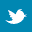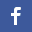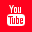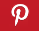Completely Solved C, C++ Programs Assignment.Quick Search Database Videos Tutorials Ebooks Forums FAQ Aboutus Household Industrial Manufacturing Service Shopping Transportation### C program to sort all the elements in a column of a 2D matrix in ascending order.

 Filed Under: C Assignments

PROBLEM STATEMENT:
Write a C program to sort all the elements in a column of a 2D matrix in ascending order.

THEORY:
In the 2D array the elements are stored in row-major form. To traverse the array, column-major wise, the variable pointing to the column is kept constant and the variable pointing to the row is incremented. In this way the columns are traversed one by one and the content of each column is sorted using the Bubble Sort technique accordingly. The variable pointing to the column is incremented and the one pointing to the row is re-initialized with 0, to start of operations with a new column.

ALGORITHM:
Begin
Read r //Size of row
Read c //Size of column
Allocate row and column sizes to array
Print ‘Enter array elements’
for iß0 to less than r, increment i
for(jß0 to less than c, increment j
Read a[i][j]
Print ’The array is ‘
for iß0 to less than r, increment i by 1
for jß0 to less than c, increment j by 1
Print a[i][j]
Newline
//Sorting columns
for iß0 to less than c, increment i by 1 //Loop to traverse through different columns
for jß0 to less than r, increment j by 1 //Bubble sorting technique
for kß0 to less than (r-1), increment k by 1
//Swapping elements
if a[k][i] is greater than a[k+1][i]
tempßa[k][i]
a[k][i]ßa[k+1][i]
a[k+1][i]temp

Print ‘The array with sorted column’
for iß0 to less than r, increment i by 1
for jß0 to less than c, increment j by 1
Print a[i][j]
Newline
End

PROGRAM LISTING:
//Program to sort columns of a given 2D matrix

#include<stdio.h>
#include<conio.h>
#include<malloc.h>

void main()
{
int **a,r,c,i,j,k,temp;
clrscr();
//Accepting row and column sizes from user
printf("Enter size of row n");
scanf("%d",&r);
printf("Enter size of column n");
scanf("%d",&c);

//Allocating memory to array
a=(int **)malloc(r*sizeof(int *));
for(i=0;i<r;i++)
a[i]=(int *)malloc(c*sizeof(int));

printf("Enter array elements n");
//Accepting array elements from user
for(i=0;i<r;i++)
for(j=0;j<c;j++)
scanf("%d",&a[i][j]);

printf("The array is : n");
//Displaying inputted array
for(i=0;i<r;i++)
{
for(j=0;j<c;j++)
printf("%d ",a[i][j]);
printf("n");
}

//Sorting columns
for(i=0;i<c;i++) //Loop to traverse through different columns
{
for(j=0;j<r;j++) //Bubble sorting technique
{
for(k=0;k<(r-1);k++)
{
if(a[k][i]>a[k+1][i])
{
//Swapping elements
temp=a[k][i];
a[k][i]=a[k+1][i];
a[k+1][i]=temp;
}//end of if
}//end of loop k
}//end of loop j
}//end of loop i

//Printing array with columns sorted
printf("The array with sorted column : n");

for(i=0;i<r;i++)
{
for(j=0;j<c;j++)
printf("%d ",a[i][j]);
printf("n");
}//end of loop i
}//end of main

OUTPUT:
Enter size of row
4
Enter size of column
4
Enter array elements
12
25
35
27
56
55
41
24
34
39
89
75
45
14
35
65
The array is :
12 25 35 27
56 55 41 24
34 39 89 75
45 14 35 65
The array with sorted column :
12 14 35 24
34 25 35 27
45 39 41 65
56 55 89 75

DISCUSSION:
The outer loop in the code to sort the elements of the array is meant for changing the index of the column after each column has been sorted.
The elements of the array can be swapped by summation followed by the subtraction technique instead of using a temporary variable.
The columns can be swapped using any kind of sorting algorithm and not just the bubble sort technique.Back to main directory:Software Practical

 @import url(http://www.google.com/cse/api/branding.css);